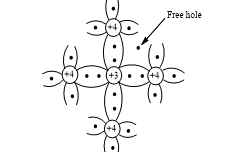## ELECTRONICS-QUIZ 11

As per analysis for previous years, it has been observed that students preparing for NEET find Physics out of all the sections to be complex to handle and the majority of them are not able to comprehend the reason behind it. This problem arises especially because these aspirants appearing for the examination are more inclined to have a keen interest in Biology due to their medical background. Furthermore, sections such as Physics are dominantly based on theories, laws, numerical in comparison to a section of Biology which is more of fact-based, life sciences, and includes substantial explanations. By using the table given below, you easily and directly access to the topics and respective links of MCQs. Moreover, to make learning smooth and efficient, all the questions come with their supportive solutions to make utilization of time even more productive. Students will be covered for all their studies as the topics are available from basics to even the most advanced. .

Q1. If a full wave rectifier circuit is operating from 50Hz mains, the fundamental frequency in the ripple will be
•  70.7 Hz
•  100 Hz
•  25 Hz
•  59 Hz
Solution
(b) For full wave rectifier, ripple frequency = 2× input frequency = 2 × 50= 100 Hz

Q2.In a cubic unit cell of bcc structure, the lattice points (ie, number of atoms) are
•  2
•  6
•  8
•  12
Solution
(a) Number of lattice points in a crystal structure will be n=Nc/8+Nf/2+Ni/1 In bcc crystal, Nc=8,Nf=0 and Ni=1 n=8/8+0/1+1/1=2

Q3. The output in the circuit of figure is taken across a capacitor. It is as shown in figure

Solution
(c) As RC time constant of the capacitor is quite large (τ=RC=10×103×10×10-6=0.1 s), it will not discharge appreciably. Hence voltage remains nearly constant

Q4. Two identical capacitors A and B are charged to the same potential V and are connected in two circuits at t=0, as shown in figure. The charge on the capacitors at time t=CR are respectively

•  VC,VC
•  VC/e,VC
•  VC,VC/e
•  VC/e,VC/e
Solution
(b) Time t=CR is known as time constant. It is time in which charge on the capacitor decreases to 1/e times of it’s initial charge (steady state charge). In figure (i) PN junction diode is in forward bias, so current will flow the circuit i.e., charge on the capacitor decrease and in time t it becomes Q=1/e (Q_o ); where Q_o=CV⇒Q=CV/e In figure (ii) P-N junction diode is in reverse bias, so no current will flow through the circuit hence change on capacitor will not decay and it remains same, i.e.,CV after time t

Q5.If De , Db and Dc are the doping levels of emitter, base and collector respectively of a transistor, then
•  De = Db = Dc
•  De < Db = Dc
•  De > Db > Dc
•  De > Dc > Db
Solution
(d) In transistor emitter is heavily doped and base is lightly doped. So, De > Dc > Db

Q6. The saturation current in a diode valve is governed by
•  Child’s law
•  Len’s law
• Richardson’s law
•  Ampere’s law
Solution
(c) For a triode, the relation is μ=rp×gm μ= amplification factor rp= plate resistance gm= mutual conductance

Q7.Which of the following does not vary with plate or grid voltages
•  gm
•  Rp
•  μ
•  Each of them varies
Solution
(d)Each of them varies

Q8.A zener diode has a contact potential of 1 V in the absence of biasing. It undergoes Zener breakdown for an electric field of 106 V-m^(-1) at the depletion region of p-n junction. If the width of the depletion region is 2.5 μm, what should be the reverse biased potential for the Zener breakdown to occur?
•  3.5 V
•  2.5 V
•  1.5 V
•  0.5 V
Solution
(b) Reverse biased potential for the zener breakdown Vr=Ed=106×2.5×10-6=2.5 volt

Q9.In order to prepare a p-type semiconductor, pure silicon can be doped with
•  Phosphorus
•  Aluminium
•  Antimony
•  Germanium
Solution
(b) When an impurity atom with 3 valence electrons (as aluminium) is introduced in a pure silicon crystal, all the three of its valence electrons form covalent bonds with one each valence electrons of the nearest
silicon atom while the valence electron of the fourth nearest silicon atom is not able to form the bond, leading to formation of hole or p-type semiconductor. While phosphorus being a pentavalent impurity leads to formation of n-type semiconductor. class="separator" style="clear: both; text-align: center;">## Want to know more

Please fill in the details below:

## Latest IITJEE Articles$type=three$c=3$author=hide$comment=hide$rm=hide$date=hide$snippet=hide ## Latest NEET Articles$type=three$c=3$author=hide$comment=hide$rm=hide$date=hide$snippet=hide

Name

ltr
item
BEST NEET COACHING CENTER | BEST IIT JEE COACHING INSTITUTE | BEST NEET & IIT JEE COACHING: ELECTRONICS-QUIZ 11
ELECTRONICS-QUIZ 11
https://1.bp.blogspot.com/-nNYQZrJBCGY/X70HQR4_yII/AAAAAAAAEY8/XkqD6CStqWQoholbL5vqHoxTQs12OJ_TgCLcBGAsYHQ/s320/Quiz%2BImage%2BTemplate%2B%252810%2529.jpg
https://1.bp.blogspot.com/-nNYQZrJBCGY/X70HQR4_yII/AAAAAAAAEY8/XkqD6CStqWQoholbL5vqHoxTQs12OJ_TgCLcBGAsYHQ/s72-c/Quiz%2BImage%2BTemplate%2B%252810%2529.jpg
BEST NEET COACHING CENTER | BEST IIT JEE COACHING INSTITUTE | BEST NEET & IIT JEE COACHING
https://www.cleariitmedical.com/2020/11/ELECTRONICS-QUIZ%2011.html
https://www.cleariitmedical.com/
https://www.cleariitmedical.com/
https://www.cleariitmedical.com/2020/11/ELECTRONICS-QUIZ%2011.html
true
7783647550433378923
UTF-8